## Trellis-oriented lexicodes

We now consider a different generating mapping for the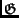-construction which will generate families of codes oriented towards a reduced decoding trellis.

There is a unique, minimal  trellis for an arbitrary linear block code. Known as the BCJR  trellis, it has no more edges or vertices at each time index than any other trellis for the code and can be constructed fairly easily [8,11]. It is shown in [8,11] that the minimal span generator matrix (MSGM) for a linear code, in which the sum of the spans of the binary generators is minimized, reflects the properties of the corresponding BCJR trellis. Namely, if G is an MSGM of a code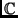, comprised of the generators G1,..., Gk, then the number of vertices Vi and edges Ei in the corresponding BCJR trellis is given by: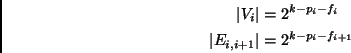(6)

where pi and fi are the dimensions of the past and future subcodes for the i-th index of. These dimensions may be computed for i = 0, 1,..., n as follows :

 pi = |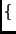j : R(Gj)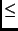i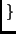|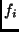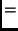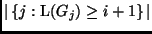As before,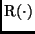and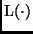refer to the rightmost and leftmost non-zero indices of a vector, respectively. With a minor modification of the lexicographic construction we may exploit the above relations to locally minimize two measures of trellis complexity: state complexity, which is the maximum number of states at any time interval of the trellis, and Viterbi decoding complexity. Specifically,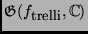is such a family of codes, and it locally minimizes trellis complexity if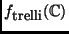returns the vector with maximum distance fromwhose bit-wise reverse ( REV) is lexicographically earliest: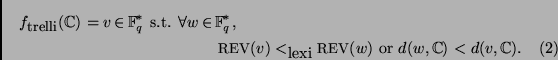For example, if the vectors {1011, 1101, 1110} are at maximum distance from a code, thenreturns the vector 1110. Because of their locally minimal trellis complexity, these codes may be justly called trellis-oriented.''

Theorem 2 establishes that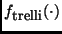locally minimizes trellis complexity among local extensions with optimal code parameters. It is possible to improve Viterbi decoding complexity by using a generating mapping which produces a longer extension, but the information rate of the resulting code will be inferior.

Theorem 2   Letbe a linear code. Among those single-iteration extensions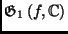with shortest length, the generator mappingminimizes the Viterbi decoding complexity and trellis state complexity.

Proof: Suppose that G is an MSGM for the (n, k, d ) codewhose past and future subcodes have dimensions pi and fi respectively, and whose trellis has vertices Vi and edges Ei, i + 1 at the i-th time interval. Now, consider appending to G the generator v =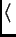1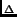|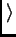as in Equation (3) of the definition of the-construction. The generated codewill have parameters (n' = n +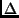, k + 1, d ). Without loss of generality we may assume that the resulting generator matrix of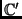is an MSGM. If we denote the difference in lengths betweenandbyn = n' - n, then a simple analysis shows thatwill have past and future subcode dimensions:

 p'i =arrayll$0$ pi -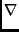n ifn < i < R(v) pi -n + 1 ifR(v)in' f'i =arrayll$k+1$ k if 0 < in fi -n ifn < in'
(7)

We may then use (7) and (9) at each time unit i to compute the number of vertices and edges ( | V'i|,| E'i|) in the BCJR trellis forbased on the vertices and edges ( | Vi|,| Ei|) in the BCJR trellis for:

 (| V'i|,| E'i|) =arrayll(1, 2) (2, 2) (2| Vi -n|, 2| Ei -n|) (| Vi -n|,| Ei -n|)
(8)

It is now a simple matter of algebra to compute the difference in Viterbi decoding complexities between the trellises ofand: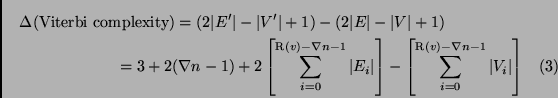Since | Ei|| Vi| for all i, minimizing the change in Viterbi decoding complexity depends on minimizing R(v) = R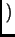1|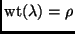, which in turn depends on having the 1 bits ofas far to the left as possible. On the other hand,cannot have weight less than the covering radius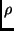ofif it is to produce an extension of shortest length. Thus, the mappingis one of several mappings which meet both criteria for local optimality: minimizing R(v) and having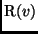. Other intuitive generating mappings, such as picking lexicographically latest vectors at maximum distance from the code, are not always locally optimal because the lexicographically latest vector need not (and generally does not) have the minimum rightmost index.

Equation 10 also proves that minimizingis the appropriate criterion for minimizing state complexity. This is because larger values ofcorrespond to doubling more vertices | Vi -n| when generating Vi'. Thus,locally minimizes state complexity as well.

width4pt depth2pt height6pt

Table: Generator matrix for the dimension 4 minimum distance 3 trellis-oriented-code. The generator padding for each iteration is marked in bold.
 0000111 0011100 0110010 1111000

Table 2 depicts the (7, 4, 3) trellis-oriented-code that was generated similarly to the code in Table 1. In fact, Method 1 can be trivially reversed to compute, without affecting the running time or space:

Method 2   Consider a set of vectors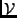, and a codewith MSGM G whose generators are in lexicographically increasing order. The following greedy method computes the lexicographically earliest bitwise reversed vector among the represented cosets in O(n| V|) time and O(n) space.


1. for each
v = (v1, v2, v3,..., v|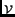|)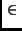do

2. 		 		fori from 1 to n

3. 		 		 		if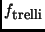is a 1 then

4.
vv + Gi

5. 		 		store the modified v;

6. 		 among all stored v, return the lexicographically earliest



The proof of correctness and complexity for Method 2 follows trivially from the proof of Method 1.